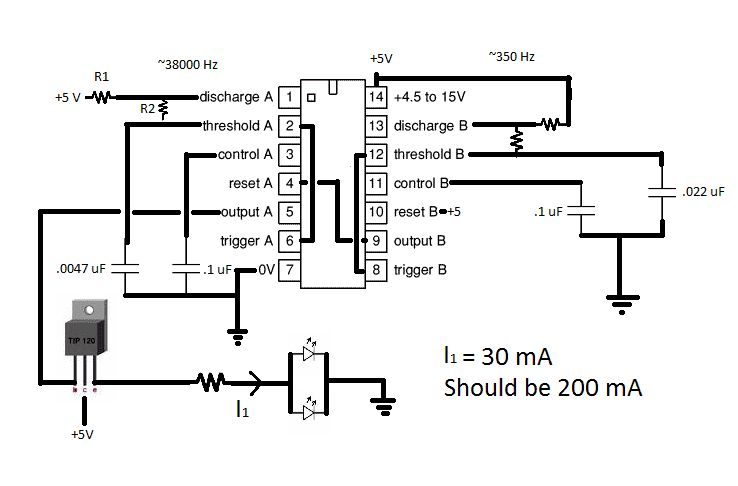# Transistor Question

jbord39
What would be the best configuration for amplifying output from 555 timer chip? No matter what transistor configuration I cannot get it higher than 30 mA (I am shooting for 200 mA).

Thanks

skeptic2
You should be able to get more than 30 mA, even 200 mA depending on the transistor, voltage and load. If you post your schematic, maybe we can help you.

Both common emitter and common collector may work. Common emitter will invert the voltage from the 555 timer. Common collector will result in a maximum output voltage slightly less than the output voltage of the 555.

jbord39
Thanks. Here is my schematic; I cannot remember resistor values, but the 556 output is 350 Hz and 38000 Hz.

Johnskeptic2
What value is the resistor between the transistor emitter and the LEDs? I think the problem is the use of a 5 V supply. Is there any reason you can't use a higher voltage?

jbord39
Would switching to maybe 9V change the frequency of both oscillators?

The value of the resistor b/w the TIP120 and the LED's (Which have Vf = 1.2V) is .5 ohms. I gradually decreased it from like 50 ohms trying to get more current but I cannot. (Minimum ~ 15 mA, maximum ~ 30 mA).

Thanks,

John

Edit: Maybe the transistor's base is at a lower voltage (due to voltage lost inside the 556?) then the +5V collector voltage. Could this cause the TIP120 to operate in the triode region rather than constant current? If so, would applying 9V to the collector instead of 5V increase the current? I am getting power from a 9V battery which is regulated to 5V with the LM317; so it would be easy to connect the collector to +9V instead of +5V.

skeptic2
Would switching to maybe 9V change the frequency of both oscillators?
Maybe the transistor's base is at a lower voltage (due to voltage lost inside the 556?) then the +5V collector voltage.
Yes and not only that, you're going to lose another ~0.65V through the transistor.

Could this cause the TIP120 to operate in the triode region rather than constant current? If so, would applying 9V to the collector instead of 5V increase the current? I am getting power from a 9V battery which is regulated to 5V with the LM317; so it would be easy to connect the collector to +9V instead of +5V.
I wouldn't say the transistor is operating in either the triode region or constant current mode. In this case the current is determined by the amount of current supplied by the 556 and the beta of the transistor, provided there is enough voltage available. It is also possible that the beta of the transistor is low and the 556 can't supply enough current. I don't think just connecting the collector to 9V will help unless you also connect the 556 to 9V too.

jbord39

My problem is that the circuit is already soldered, so I was wondering how much you think changing the supply voltage from regulated +5 to 9V battery would change the output frequencies of the timers.

Thanks,

John

skeptic2
Changing the voltage will change the frequency very slightly, probably much less than the tolerance of your components. I'd be surprised if it shifted the frequency so much that your detector stopped detecting it.

It depends on how you measure it, too.

If you are just using a meter to measure it, you will get a reading of average current.
But in this case the duty cycle will be about 25% or so.

You have a square wave turning on and off at 38 KHz, so that probably has a duty cycle of about 50%, and then you are turning this square wave on and off with a 350 Hz square wave. So, the duty cycle could be something like 25%, or maybe even less.

So, to get a current reading of 30 mA, the actual current might be more like 120 mA when it is flowing through the LEDs.

jbord39
I am pretty sure my DMM is made to measure square wave currents; but to make sure I also monitored the voltage across the resistor with an oscilloscope, and it was only getting 30 mA. I ended up getting 200 mA using the transistor configuration though.

Thanks guys,

John